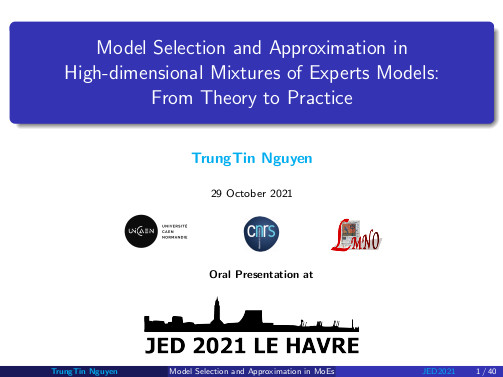# Model Selection and Approximation in High-dimensional Mixtures of Experts Models From Theory to PracticeImage credit: TrungTin Nguyen

### Abstract

Mixtures of experts (MoE) models are a ubiquitous tool for the analysis of heterogeneous data across many fields including statistics, bioinformatics, pattern recognition, economics, and medicine, among many others. They provide conditional constructions for regression in which the mixture weights, along with the component densities, are explained by the predictors, allowing for flexibility in the modeling of data arising from complex data generating processes. In this thesis, we study the approximation capabilities and model estimation and selection properties, of a wide variety of mixture distributions, with a particular focus on a rich family of MoE models in a high-dimensional setting, including MoE models with Gaussian experts and softmax or Gaussian gating functions, which are the most popular choices and are powerful tools for modeling complex non-linear relationships between responses and predictors that arise from different subpopulations. We consider both the theoretical statistical and methodological aspects, and the numerical tools, related to the conception of these models, as well as to their data-driven estimation and model selection. More precisely, in this thesis, we first review the universal approximation properties of classical mixture distributions in order to prepare the theoretical framework and to clarify some unclear and vague statements in the literature, before considering them in the context of MoE models. In particular, we prove that, to an arbitrary degree of accuracy, location-scale mixtures of a continuous probability density function (PDF) can approximate any continuous PDF, uniformly, on a compact set; and location-scale mixtures of an essentially-bounded PDF can approximate any PDF in Lebesgue spaces. Then, after improving upon approximation results in the context of unconditional mixture distributions, we study the universal approximation capabilities of MoE models in a variety of contexts, including conditional density approximation and approximate Bayesian computation (ABC). Given input and output variables are both compactly supported, we provide denseness results in Lebesgue spaces for conditional PDFs. Moreover, we prove that the quasi-posterior distribution resulting from ABC with surrogate posteriors built from finite Gaussian mixtures using an inverse regression approach, converges to the true one, under standard conditions. Finally, we establish non-asymptotic risk bounds that take the form of weak oracle inequalities, provided that lower bounds on the penalties hold true, in high-dimensional regression scenarios for a variety of MoE regression models, including Gaussian-gated and softmax-gated Gaussian MoE, based on an inverse regression strategy or a Lasso penalization, respectively. In particular, our oracle inequalities show that the performance in Jensen–Kullback–Leibler type loss of our penalized maximum likelihood estimators are roughly comparable to that of oracle models if we take large enough the constants in front of the penalties, whose forms are only known up to multiplicative constants and proportional to the dimensions of models. Such theoretical justifications of the penalty shapes motivate us to make use of the slope heuristic criterion to select several hyperparameters, including the number of mixture components, the amount of sparsity (the coefficients and ranks sparsity levels), the degree of polynomial mean functions, and the potential hidden block-diagonal structures of the covariance matrices of the multivariate predictor or response variable. To support our theoretical results and the statistical study of non-asymptotic model selection in a variety of MoE models, we perform numerical studies by considering simulated and real data, which highlight the performance of our finite-sample oracle inequality results.

Date
Oct 29, 2021 9:00 AM — 6:00 PM
Location
Laboratoire d’Ondes et Milieux Complexes, Le Havre, France##### TrungTin Nguyen
###### Postdoctoral Fellow

A central theme of my research is data science at the intersection of statistical learning, machine learning and optimization.